Refractive Index of a Liquid

# Our Objective:

To determine the refractive index of a liquid using convex lens by parallax method.

# The Theory: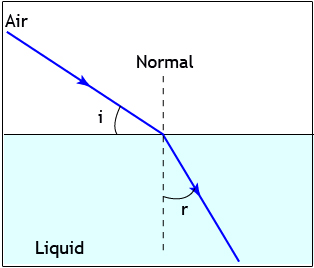In optics, the refractive index or index of refraction n of a material is a dimensionless number that describes how light propagates through that medium. It is defined as:

n = c/v

where,  c is the speed of light in vacuum and v is the phase velocity of light in the medium. For example, the refractive index of water is 1.333, meaning that light travels 1.333 times faster in a vacuum than it does in water.

The refractive index determines how much light is bent, or refracted, when entering a material. When light moves from one medium to another, it changes its direction, i.e., it is refracted.

If i is the angle of incidence of a ray in vacuum and r is the angle of refraction, the refractive index n is defined as the ratio of the sine of the angle of incidence to the sine of the angle of refraction.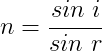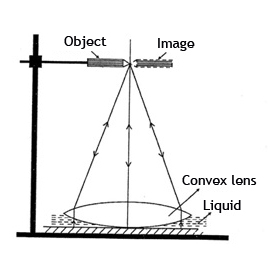When a convex lens is placed over some drops of the given liquid on a plane mirror, a plano-concave liquid lens is formed between the lens and the mirror.

If f1 is the focal length of the convex lens and F is the focal length of the combination of convex lens and plano-concave liquid lens, the focal length of the liquid lens f2 is given by,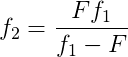If R is the radius curvature of the face of the convex lens which is in contact with the liquid, then the refractive index of the liquid is,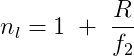# Learning outcomes:

• Students understand the terms refractive index and plano–concave liquid lens.
• Students will be better able to do the experiment in a real laboratory by understanding the procedure.